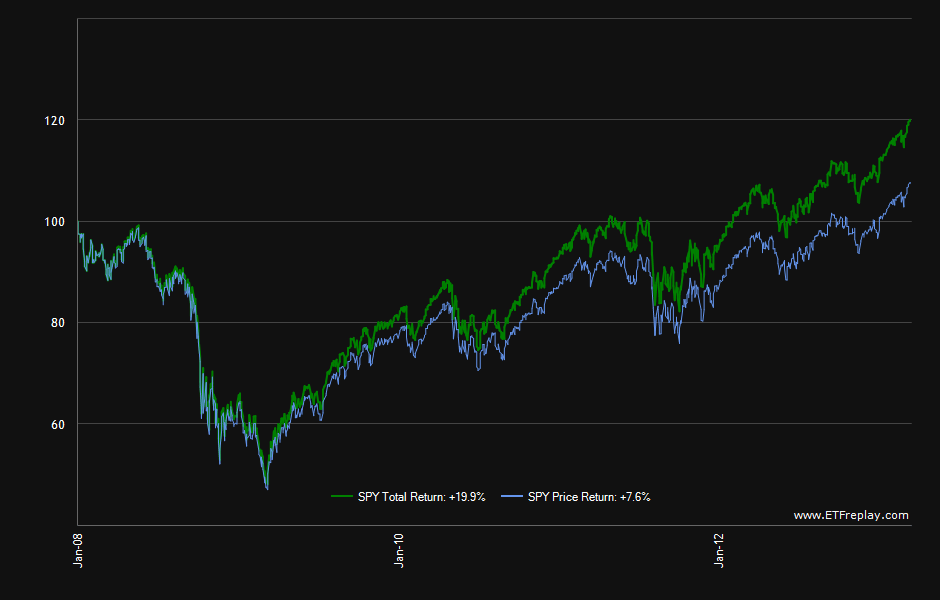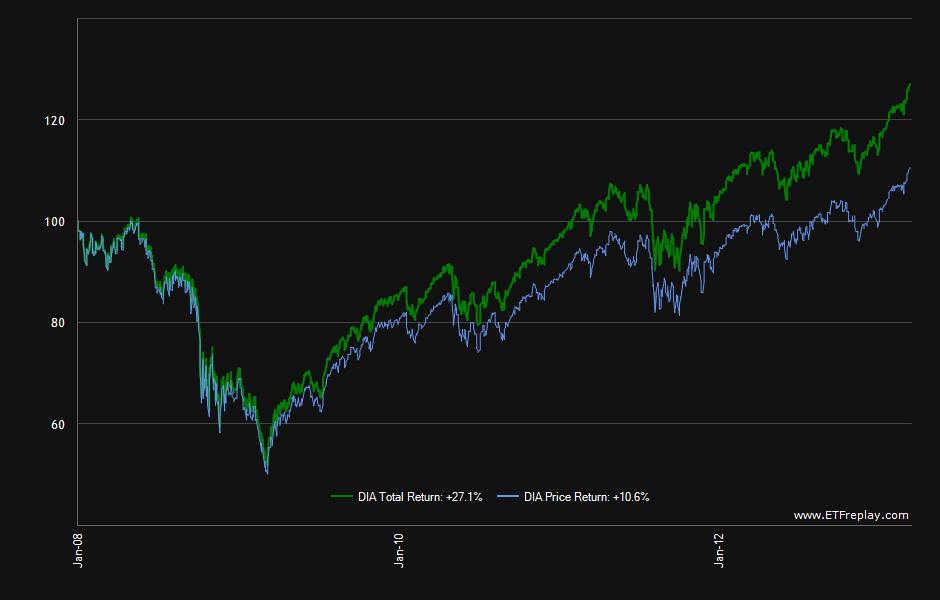# Total Return vs. Price Return of Two ETFs

Investors can the amplify returns of an investment in a stock by reinvesting dividends.This return known as the Total Return will be substantially higher than the Price Return  in the long-term  due to the effect of compounding. The difference in returns between the total return and price return will be especially significant for companies with above-average and growing dividend yields.

While this strategy works with stocks does it work with ETFs?

Since ETFs are similar to stocks in many aspects one can assume that this strategy will work with ETFs also. So in order to verify this assumption I did a quick check of returns using two of the largest ETFs for US stocks. The results show that total returns for ETFs is higher than price returns. Unlike stocks ETFs can have other distributions such as capital gains in addition to dividends. Hence the total return calculated for ETFs includes both dividends and distributions.

1)  Total Return vs. Price Return for SPDR S&P 500 ETF (SPY) from Jan 2,2008 to Mar 13, 2013:

Click to enlargeAs the chart above shows the gap between the two returns is indeed large. Reinvestment of dividends and distributions yielded 12.3% more than the return from price appreciation alone.

Currently the ETF has \$125.0 billion in assets and a 2.04% distribution yield. The annual dividend yield is 1.99%.

2) Total Return vs. Price Return for SPDR Dow Jones Industrial Average ETF (DIA) from Jan 2,2008 to Mar 13, 2013:Source: ETFreplay.com

The total return for this ETF is more than double that of the price return for the period shown.

Currently  the total assets of this fund is \$10.0 billion and the distribution yield is 2.36%.The annual dividend yield is 2.29%.

Note: Distribution yields and asset sizes noted are as of Mar 13, 2013. Data is known to be accurate from sources used. Please do your own research before making any investment decisions.

Disclosure: No Positions